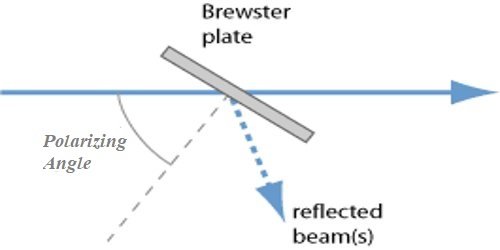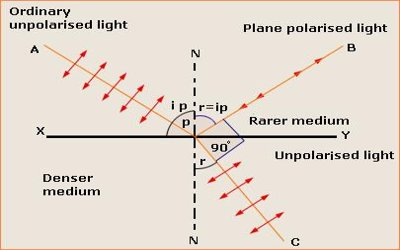# Polarizing Angle

Polarizing Angle: In a reflecting medium, if the angle of incidence is changed slowly then an angle will be obtained for which the polarization will be maximum, that angle is called the polarizing angle. It is the angle at which unpolarized light or other electromagnetic radiation must be incident upon a nonmetallic surface for the reflected radiation to acquire maximum plane polarization. When unpolarized light is incident at this angle, the light that is reflected from the surface is therefore perfectly polarized.

The polarization of light is the direction of the electric field component. Polarization angle is the angle between electric field vector and incidence plane. Incidence plane is all incident, reflected and refracted beams lie in. The angle of incidence (differing for different substances) at which a ray of light incident on a surface is polarized to the greatest degree on reflection at the surface.Fig: Polarizing Angle

Polarizing angle or Brewster’s angle can be calculated using the following equation:

n = sin(qi)/sin(qr) = sin(qi)/sin(q90-i) = tan(qi)

where n is the refractive index of the medium of light reflection, qi is the angle of incidence, and qr is the angle of refraction.

This equation is also useful when determining the refractive index of an unknown specimen, such as opaque materials with high absorption coefficients for light transmission.